# Chemical Equilibrium Reversible Reactions Most chemical reactions are

• Slides: 42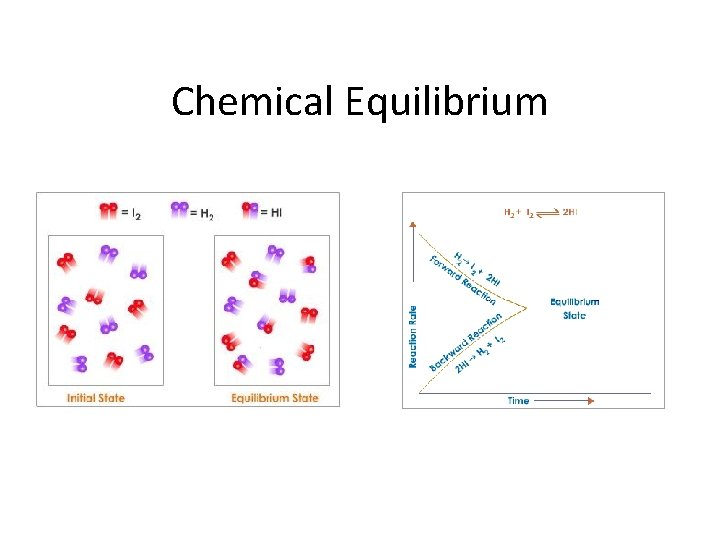Chemical Equilibrium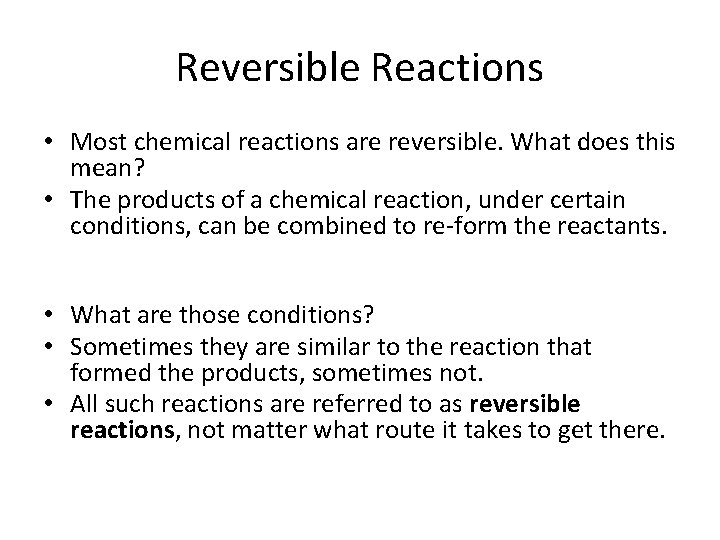Reversible Reactions • Most chemical reactions are reversible. What does this mean? • The products of a chemical reaction, under certain conditions, can be combined to re-form the reactants. • What are those conditions? • Sometimes they are similar to the reaction that formed the products, sometimes not. • All such reactions are referred to as reversible reactions, not matter what route it takes to get there.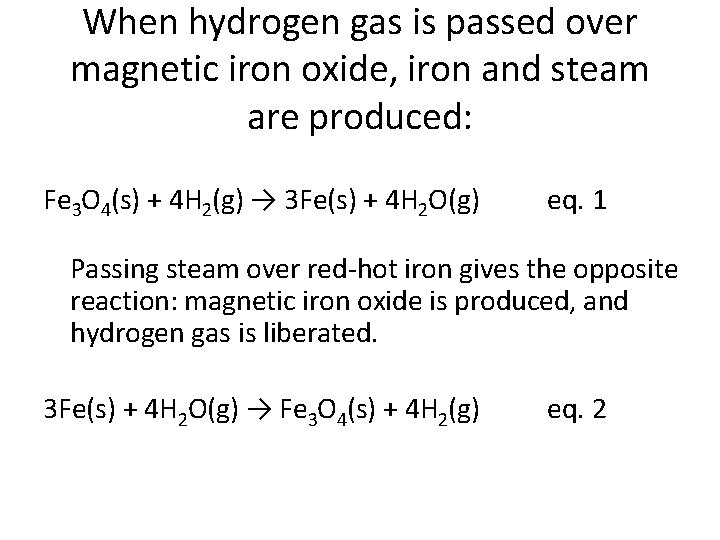When hydrogen gas is passed over magnetic iron oxide, iron and steam are produced: Fe 3 O 4(s) + 4 H 2(g) → 3 Fe(s) + 4 H 2 O(g) eq. 1 Passing steam over red-hot iron gives the opposite reaction: magnetic iron oxide is produced, and hydrogen gas is liberated. 3 Fe(s) + 4 H 2 O(g) → Fe 3 O 4(s) + 4 H 2(g) eq. 2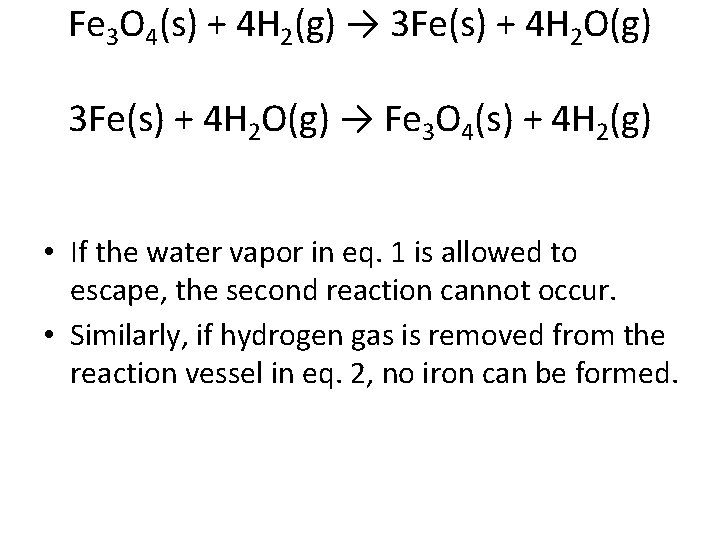Fe 3 O 4(s) + 4 H 2(g) → 3 Fe(s) + 4 H 2 O(g) → Fe 3 O 4(s) + 4 H 2(g) • If the water vapor in eq. 1 is allowed to escape, the second reaction cannot occur. • Similarly, if hydrogen gas is removed from the reaction vessel in eq. 2, no iron can be formed.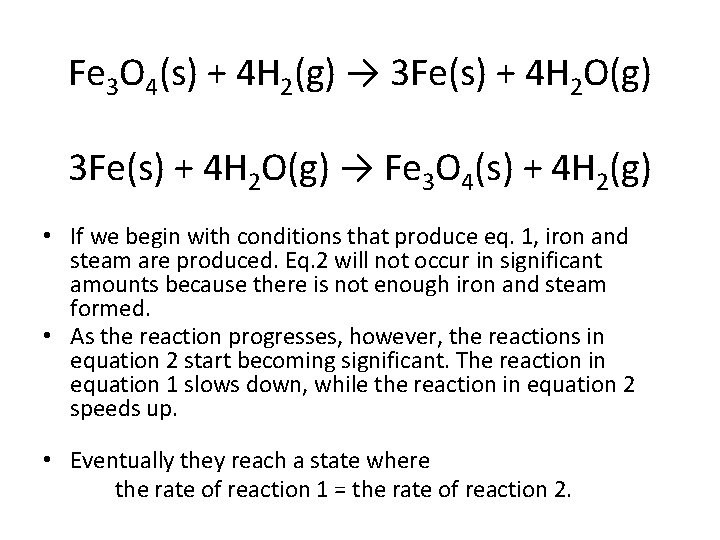Fe 3 O 4(s) + 4 H 2(g) → 3 Fe(s) + 4 H 2 O(g) → Fe 3 O 4(s) + 4 H 2(g) • If we begin with conditions that produce eq. 1, iron and steam are produced. Eq. 2 will not occur in significant amounts because there is not enough iron and steam formed. • As the reaction progresses, however, the reactions in equation 2 start becoming significant. The reaction in equation 1 slows down, while the reaction in equation 2 speeds up. • Eventually they reach a state where the rate of reaction 1 = the rate of reaction 2.Chemical Equilibrium • When this happens, the system is said to be in chemical equilibrium: • Note that for reactions in equilibrium, arrows in both directions are used. • The substances on the left are called reactants, and the substances on the right are called products.H 2(g) +I 2(g) ↔ 2 HI(g)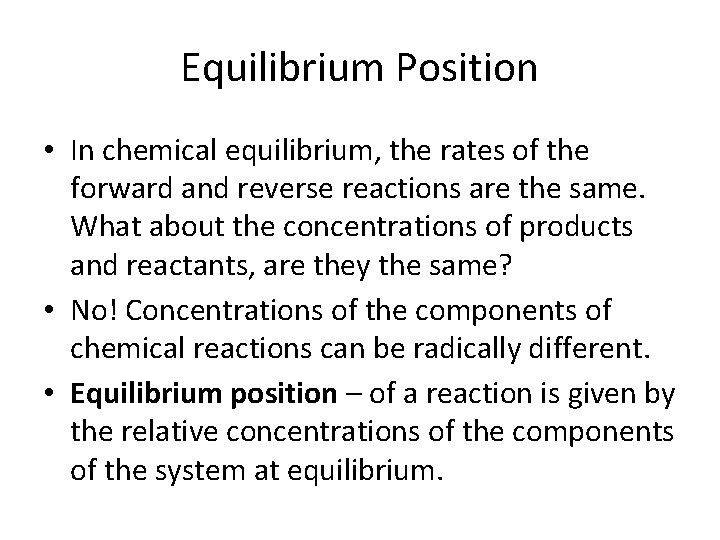Equilibrium Position • In chemical equilibrium, the rates of the forward and reverse reactions are the same. What about the concentrations of products and reactants, are they the same? • No! Concentrations of the components of chemical reactions can be radically different. • Equilibrium position – of a reaction is given by the relative concentrations of the components of the system at equilibrium.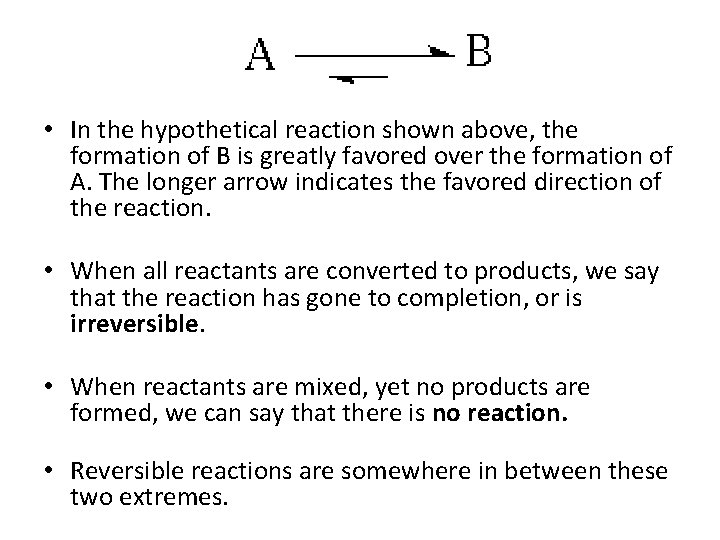• In the hypothetical reaction shown above, the formation of B is greatly favored over the formation of A. The longer arrow indicates the favored direction of the reaction. • When all reactants are converted to products, we say that the reaction has gone to completion, or is irreversible. • When reactants are mixed, yet no products are formed, we can say that there is no reaction. • Reversible reactions are somewhere in between these two extremes.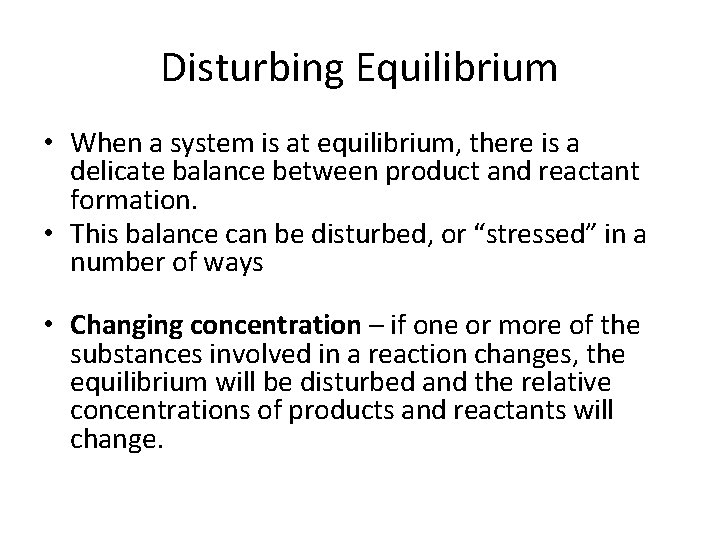Disturbing Equilibrium • When a system is at equilibrium, there is a delicate balance between product and reactant formation. • This balance can be disturbed, or “stressed” in a number of ways • Changing concentration – if one or more of the substances involved in a reaction changes, the equilibrium will be disturbed and the relative concentrations of products and reactants will change.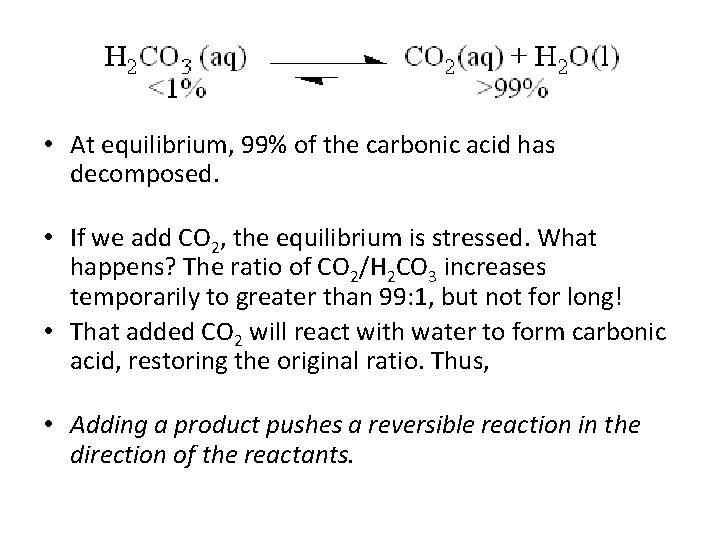• At equilibrium, 99% of the carbonic acid has decomposed. • If we add CO 2, the equilibrium is stressed. What happens? The ratio of CO 2/H 2 CO 3 increases temporarily to greater than 99: 1, but not for long! • That added CO 2 will react with water to form carbonic acid, restoring the original ratio. Thus, • Adding a product pushes a reversible reaction in the direction of the reactants.• The same process occurs if we remove CO 2 instead of adding it – initially the ratio of CO 2/H 2 CO 3 declines, but this is followed by increased decomposition of carbonic acid: • Removing a product pushes a reversible reaction in the direction of products. • These properties of equilibria are often used to increase yields of chemical reaction – products are removed as soon as they are formed, so the system is continually stressed and produces more products.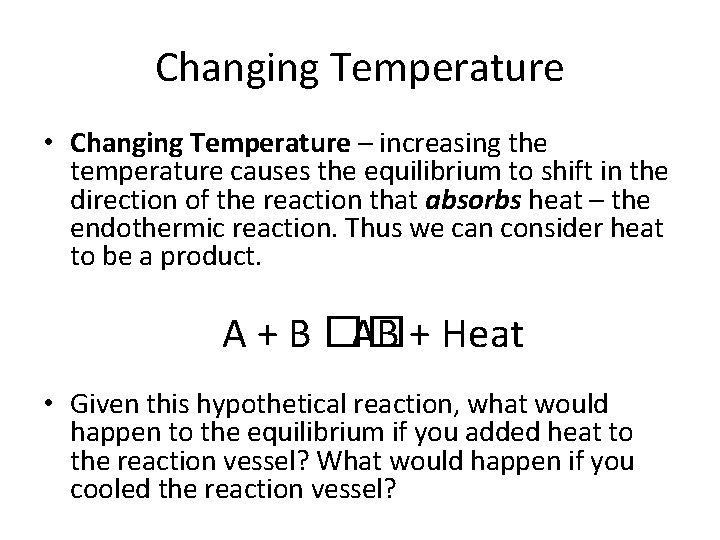Changing Temperature • Changing Temperature – increasing the temperature causes the equilibrium to shift in the direction of the reaction that absorbs heat – the endothermic reaction. Thus we can consider heat to be a product. A + B �� AB + Heat • Given this hypothetical reaction, what would happen to the equilibrium if you added heat to the reaction vessel? What would happen if you cooled the reaction vessel?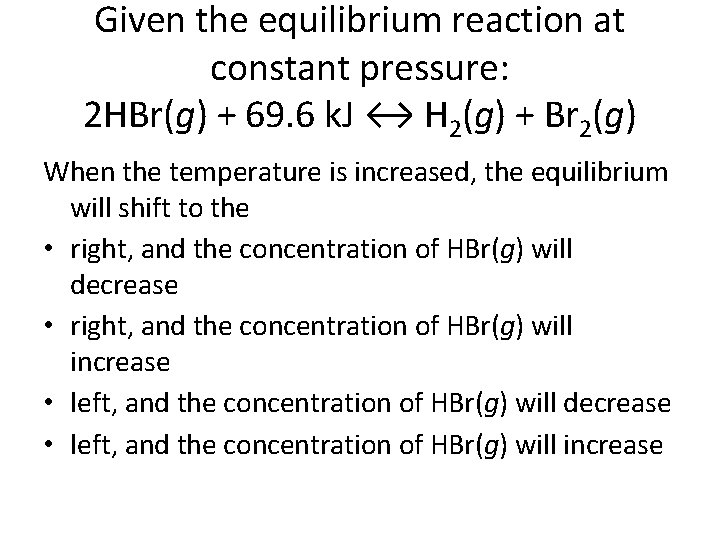Given the equilibrium reaction at constant pressure: 2 HBr(g) + 69. 6 k. J ↔ H 2(g) + Br 2(g) When the temperature is increased, the equilibrium will shift to the • right, and the concentration of HBr(g) will decrease • right, and the concentration of HBr(g) will increase • left, and the concentration of HBr(g) will decrease • left, and the concentration of HBr(g) will increase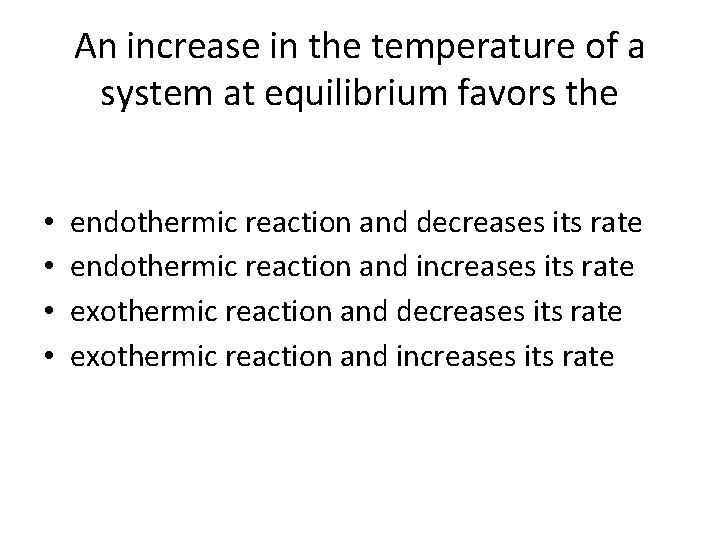An increase in the temperature of a system at equilibrium favors the • • endothermic reaction and decreases its rate endothermic reaction and increases its rate exothermic reaction and decreases its rate exothermic reaction and increases its rateChanging Pressure • For gases, a change in pressure will have no effect on the equilibrium if the reactants have the same number of molecules (moles) as the products. • However, if the number of moles of product is different from the number of moles of reactant, pressure changes have dramatic effects on equilibrium.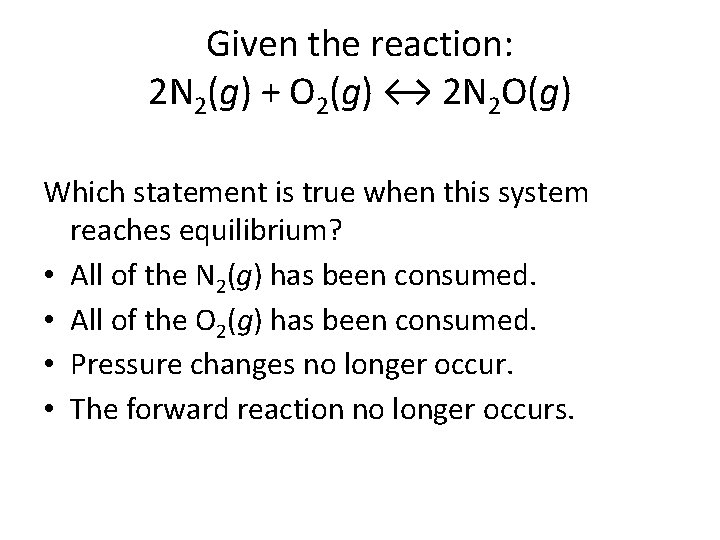Given the reaction: 2 N 2(g) + O 2(g) ↔ 2 N 2 O(g) Which statement is true when this system reaches equilibrium? • All of the N 2(g) has been consumed. • All of the O 2(g) has been consumed. • Pressure changes no longer occur. • The forward reaction no longer occurs.Given the reaction at equilibrium: 2 CO(g) + O 2(g) ↔ 2 CO 2(g) When the reaction is subjected to stress, a change will occur in the concentration of • reactants, only • products, only • both reactants and products • neither reactants nor productsA(g) + 3 B(g) ↔ 2 C(g) • Note that in this reaction, the products have half the number of moles as the reactants do. • When this reaction proceeds, the pressure is reduced. • Let’s say you have the above reaction in a container, and you reduce the pressure further. What happens? • Reducing pressure pushes the reaction towards the reactants. • To restore the original pressure, product “C” decomposes to A and B, increasing the number of molecules.Le Chatelier’s Principle • The relationships between equilibrium and concentration, temperature, and pressure were elucidated by Henri-Louis Le Chatelier at the turn of the century. • In particular, he was interested in producing ammonia from hydrogen and nitrogen: N 2(g) + 3 H 2(g) ↔ 2 NH 3(g) • Although he didn’t succeed in creating an industrial process for ammonia production, another scientist, Fritz Haber, was successful. • Le Chatelier’s work is summarized in a simple statement, Le Chatelier’s Principle.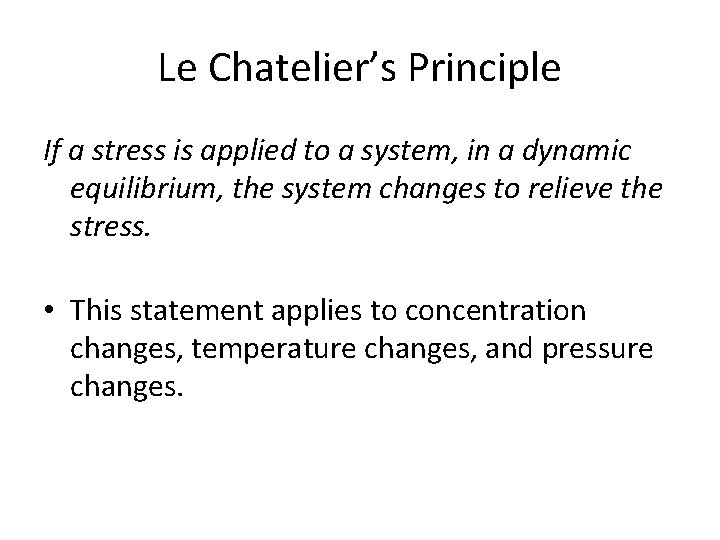Le Chatelier’s Principle If a stress is applied to a system, in a dynamic equilibrium, the system changes to relieve the stress. • This statement applies to concentration changes, temperature changes, and pressure changes.Ammonia is produced commercially by the Haber reaction: N 2(g) + 3 H 2(g) ↔ 2 NH 3(g) + energy The formation of ammonia is favored by • an increase in pressure • a decrease in pressure • removal of N 2(g) • removal of H 2(g)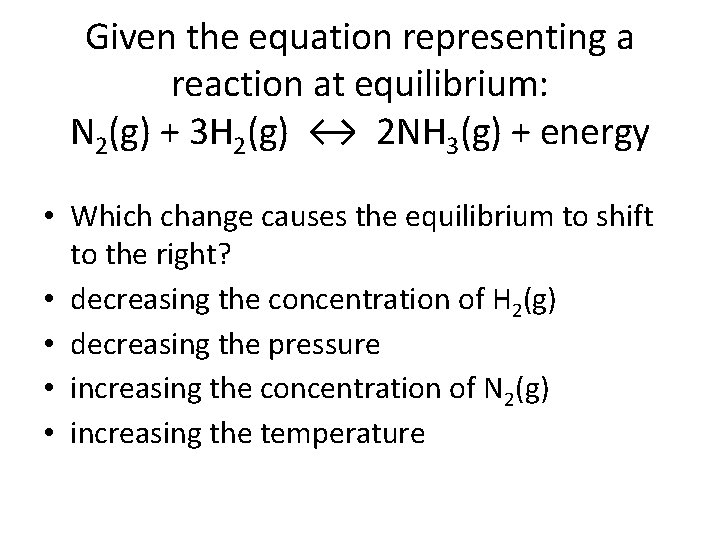Given the equation representing a reaction at equilibrium: N 2(g) + 3 H 2(g) ↔ 2 NH 3(g) + energy • Which change causes the equilibrium to shift to the right? • decreasing the concentration of H 2(g) • decreasing the pressure • increasing the concentration of N 2(g) • increasing the temperature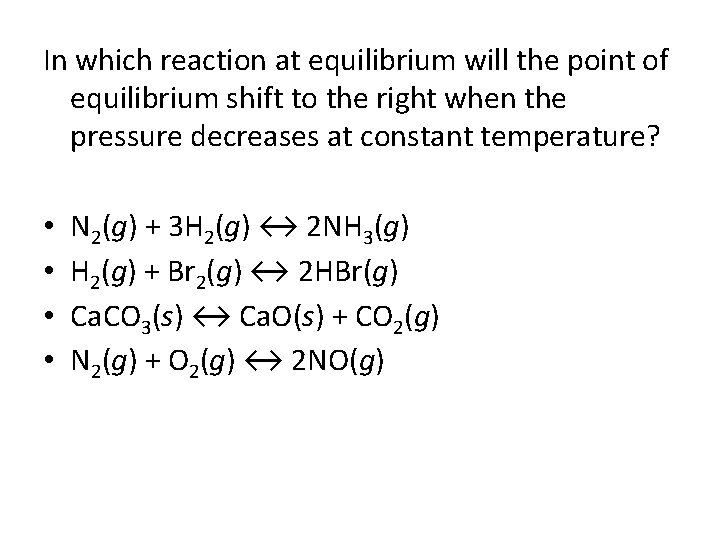In which reaction at equilibrium will the point of equilibrium shift to the right when the pressure decreases at constant temperature? • • N 2(g) + 3 H 2(g) ↔ 2 NH 3(g) H 2(g) + Br 2(g) ↔ 2 HBr(g) Ca. CO 3(s) ↔ Ca. O(s) + CO 2(g) N 2(g) + O 2(g) ↔ 2 NO(g)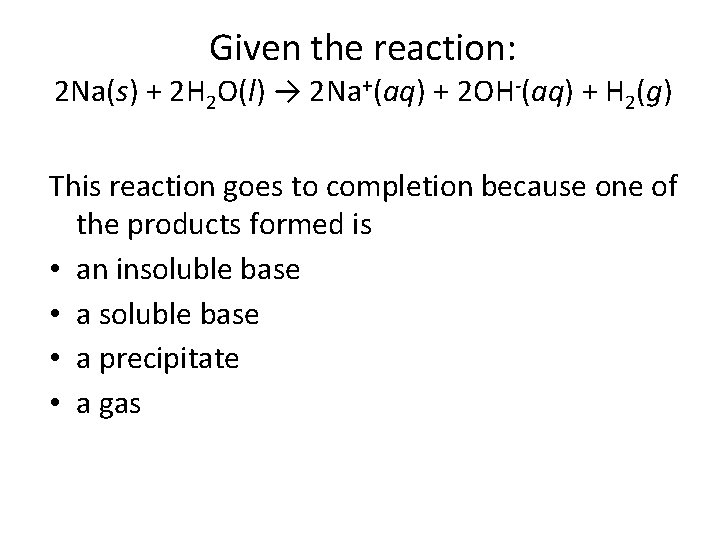Given the reaction: 2 Na(s) + 2 H 2 O(l) → 2 Na+(aq) + 2 OH-(aq) + H 2(g) This reaction goes to completion because one of the products formed is • an insoluble base • a precipitate • a gasThe Haber Process N 2(g) + 3 H 2(g) ↔ 2 NH 3(g) + 92 k. JNitrogenHaber’s Idea • At 500 C, yield is 0. 1%!! At 700 atm, yield is 47%!!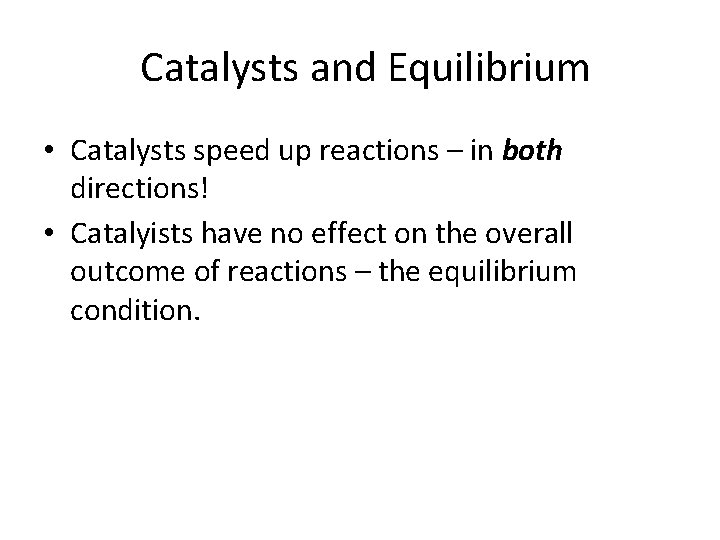Catalysts and Equilibrium • Catalysts speed up reactions – in both directions! • Catalyists have no effect on the overall outcome of reactions – the equilibrium condition.Given the reaction at equilibrium: Which change will not affect the equilibrium concentrations of A(g), B(g), and A 2 B 3(g)? • adding more A(g) • adding a catalyst • increasing the temperature • increasing the pressurePhysical Equilibria • Equilibrium also occurs in many physical processes: - saturated solutions - phase changes evaporation of liquids (vapor pressure) evaporation of solids (sublimation) condensation of gases to liquids and solids (deposition)Tables G and H Reflect Physical Equilibrium Processes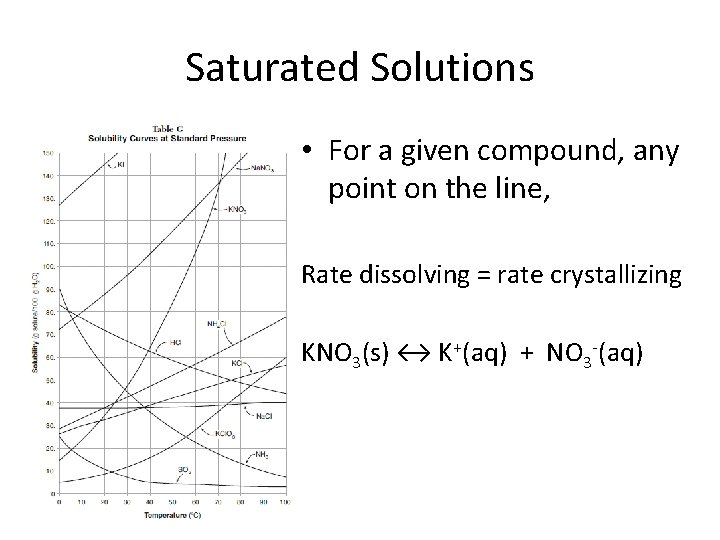Saturated Solutions • For a given compound, any point on the line, Rate dissolving = rate crystallizing KNO 3(s) ↔ K+(aq) + NO 3 -(aq)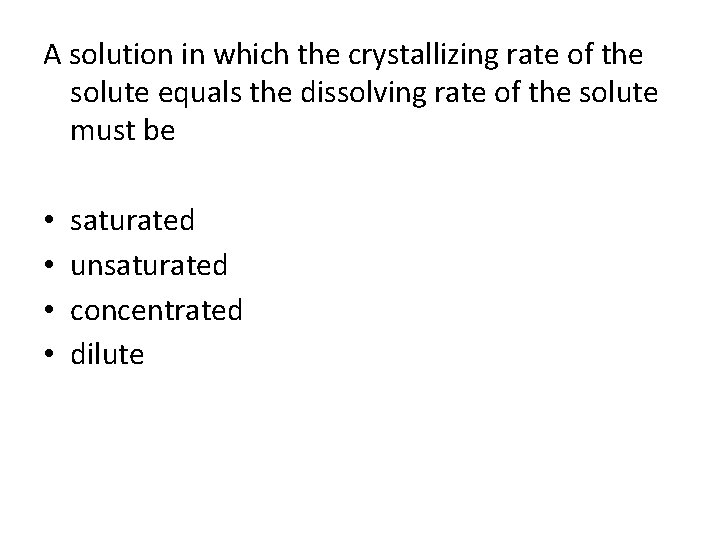A solution in which the crystallizing rate of the solute equals the dissolving rate of the solute must be • • saturated unsaturated concentrated dilute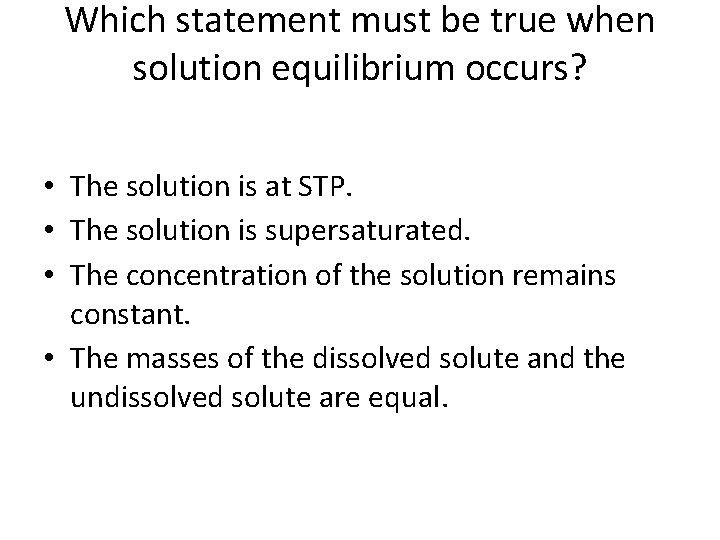Which statement must be true when solution equilibrium occurs? • The solution is at STP. • The solution is supersaturated. • The concentration of the solution remains constant. • The masses of the dissolved solute and the undissolved solute are equal.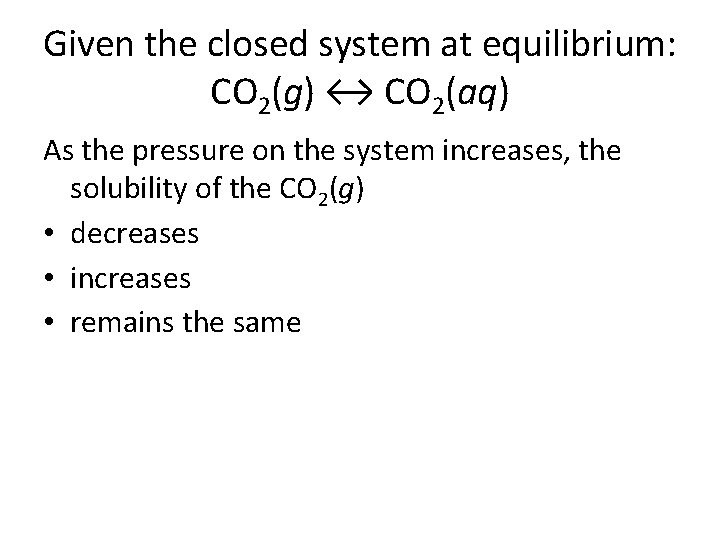Given the closed system at equilibrium: CO 2(g) ↔ CO 2(aq) As the pressure on the system increases, the solubility of the CO 2(g) • decreases • increases • remains the same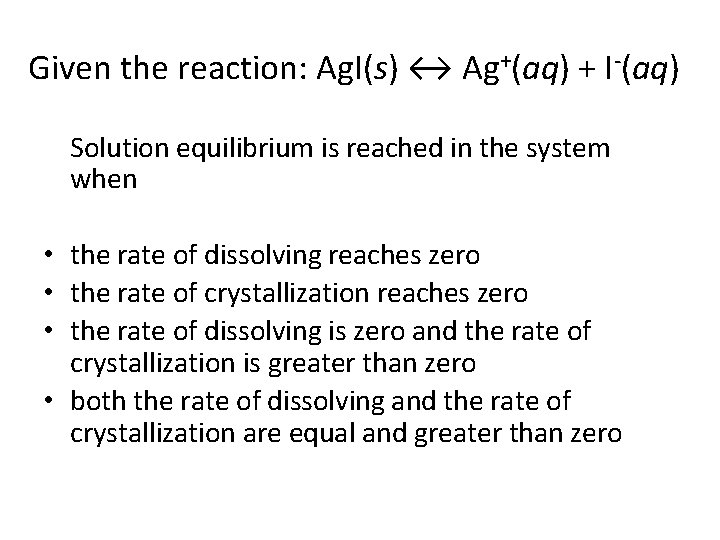Given the reaction: Ag. I(s) ↔ Ag+(aq) + I-(aq) Solution equilibrium is reached in the system when • the rate of dissolving reaches zero • the rate of crystallization reaches zero • the rate of dissolving is zero and the rate of crystallization is greater than zero • both the rate of dissolving and the rate of crystallization are equal and greater than zero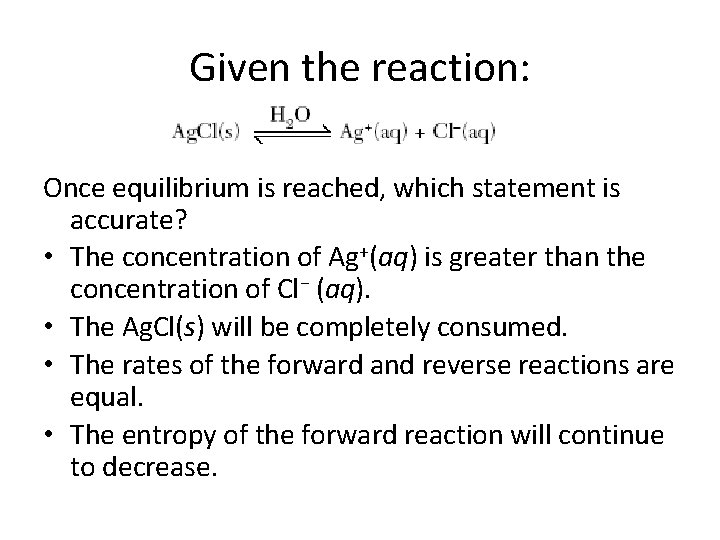Given the reaction: Once equilibrium is reached, which statement is accurate? • The concentration of Ag+(aq) is greater than the concentration of Cl− (aq). • The Ag. Cl(s) will be completely consumed. • The rates of the forward and reverse reactions are equal. • The entropy of the forward reaction will continue to decrease.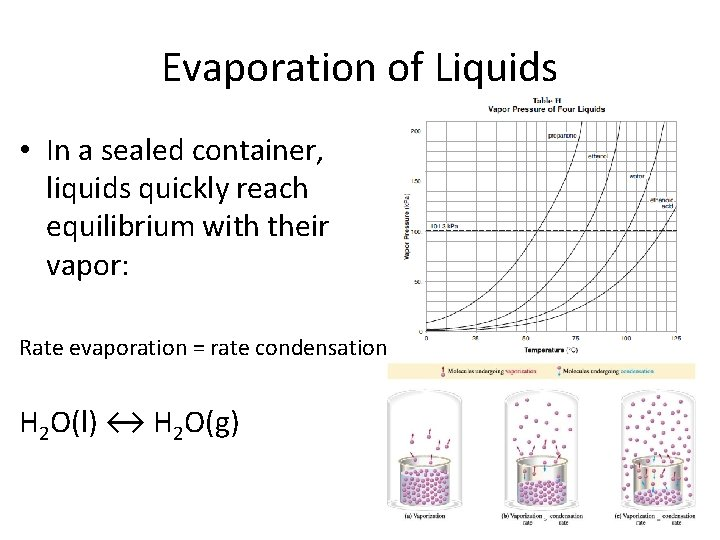Evaporation of Liquids • In a sealed container, liquids quickly reach equilibrium with their vapor: Rate evaporation = rate condensation H 2 O(l) ↔ H 2 O(g)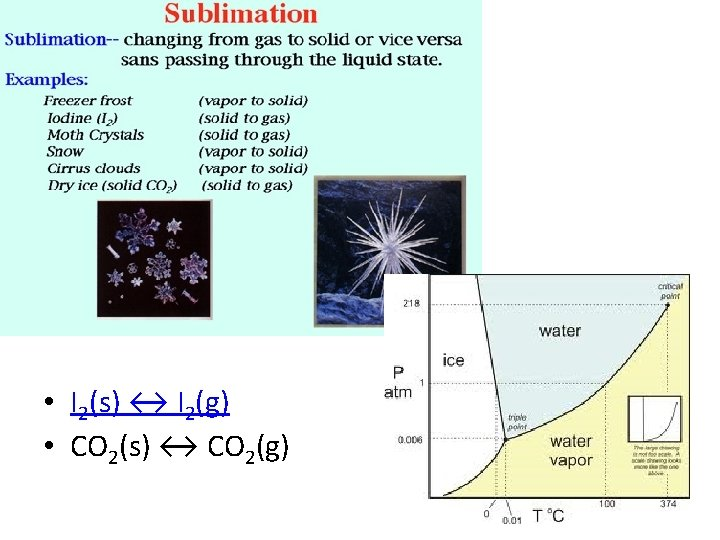Which type or types of change, if any, can reach equilibrium? • • a chemical change, only a physical change, only both a chemical and a physical change neither a chemical nor a physical changeWhich equation represents a system that will reach equilibrium in the flask? • Na. Cl(s) ↔ Na. Cl(l) • Na. Cl(s) ↔ H 2 O(l) • H 2 O(g) ↔ Na. Cl(aq) • H 2 O(g) ↔ H 2 O(l)